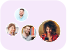Product Edition:2nd Edition
Author: Henry Ricardo, Henry J. Ricardo, Henry J Ricardo
Book Name: A Modern Introduction to Differential Equations
Subject Name: Maths

# A Modern Introduction to Differential Equations 2nd Edition Solutions

0 out of 5.0
40 reviews44 Students
have requested for homework help from this book

A Modern Introduction to Differential Equations Second Edition provides an introduction to the basic concepts of differential equations.The book begins by introducing the basic concepts of differential equations focusing on the analytical graphical and numerical aspects of first-order equations including slope fields and phase lines. The discussions then cover methods of solving second-order homogeneous and nonhomogeneous linear equations with constant coefficientssystems of linear differential equationsthe Laplace transform and its applications to the solution of differential equations and systems of differential equationsand systems of nonlinear equations. Each chapter concludes with a summary of the important concepts in the chapter. Figures and tables are provided within sections to help students visualize or summarize concepts. The book also includes examples and exercises drawn from biology chemistry and economics as well as from traditional pure mathematics physics and engineering.This book is designed for undergraduate students majoring in mathematics the natural sciences and engineering. However students in economics business and the social sciences with the necessary background will also find the text useful.Student friendly readability- assessible to the average studentEarly introduction of qualitative and numerical methodsLarge number of exercises taken from biology chemistry economics physics and engineeringExercises are labeled depending on difficulty/sophisticationEnd of chapter summariesGroup projectsRead more

5
20
4
8
3
2
2
8
1
2

0

## Students who viewed this book also checked out